Monday, September 27, 2021
Home > download jstse papers > JSTSE PREVIOUS YEAR QUESTION PAPER 2010-11

# JSTSE PREVIOUS YEAR QUESTION PAPER 2010-11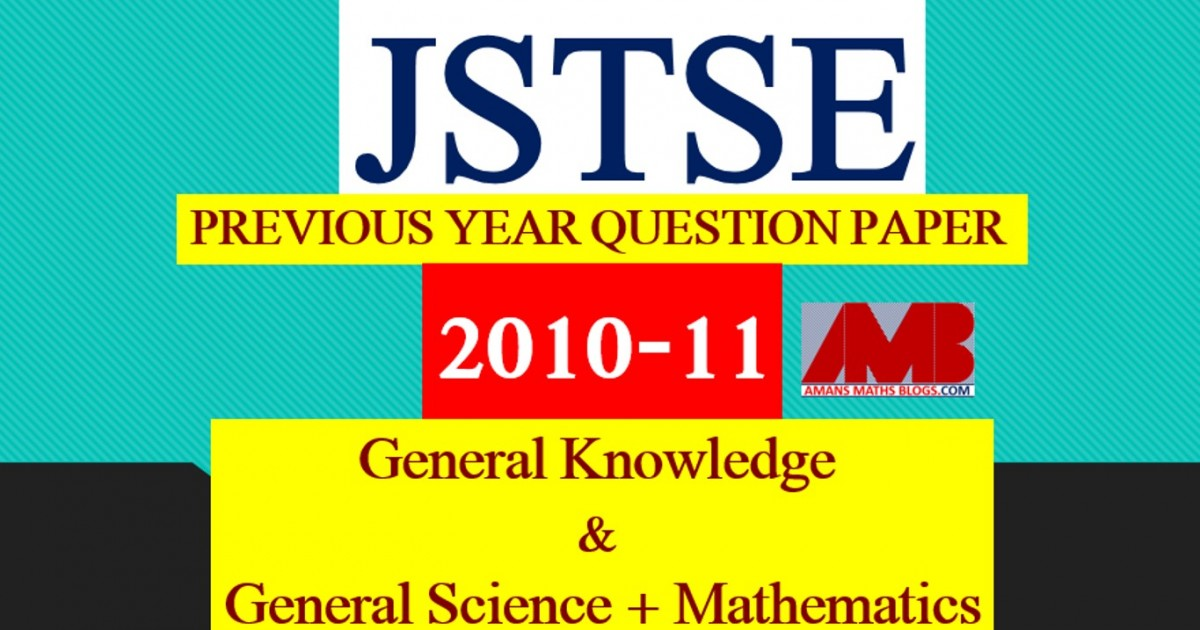# JSTSE PREVIOUS YEAR QUESTION PAPER 2010-11

Junior Science Talent Search Examination (JSTSE) is conducted by Science Branch of DTE of Education, Delhi every year usually in the month of January for the students of recognized schools of Delhi. In this post, you will get JSTSE Previous Year Question Paper 2010-11.

JSTSE
Previous Year Question Papers
2007-082008-092009-102010-112011-12
2012-132013-142014-152015-162016-17
2018-19PhysicsChemistryMathsBiology

Eligibility: – Any student of recognised school studying in Class IX in Govt./Govt. Aided/Public/KV/Navodaya/NDMC and must have secured 65% marks in class VIII.
Examination fee: – No examination fee is charged from students for appearing in this exam.
Type of Examination: – The examination consists of two papers.
I. General Knowledge
No. of Questions: 50       Marks : 50     Time Alloted: 50 min
50 50 50 min.
II . General Science and Mathematics
No. of Questions : 150      Marks : 150     Time Alloted: 150 min

No. of Scholarships:- There are 150 scholarships for JSTSE per year.

## General Science + Mathematics

JSTSE Previous Year Question Papers 2010-11 Ques No 1:

The P/Q form of 23.43 is

Options:

A. 2343/99

B. 2343/100

C. 2320/99

D. 2343/999

JSTSE Previous Year Question Paper 2010-11 Ques No 2:

If x = 1 – √2, then the value of (x – x-1)3 is

Options:

A. 2√2

B. 8

C. 1

D. None of above

JSTSE Previous Year Question Papers 2010-11 Ques No 3:

If 27x = 9/3x, then the value of x is

Options:

A. 0

B. 1/2

C. 1/3

D. 1

JSTSE Previous Year Question Paper 2010-11 Ques No 4:

On simplifying (1/2)3 + (1/3)3 – (5/6)3 we get

Options:

A. 0

B. -1

C. -5/12

D. 5/12

JSTSE Previous Year Question Papers 2010-11 Ques No 5:

If 109x/5 = 2090 x 5-1, then the value of x is

Options:

A. -1

B. 0

C. 1

D. None of above

JSTSE Previous Year Question Paper 2010-11 Ques No 6:

On simplifying (399 + 398) / (3100 – 399), we get

Options:

A. 3197

B. 3196

C. 3/2

D. 2/3

JSTSE Previous Year Question Papers 2010-11 Ques No 7:

In a triangle ABC, the internal bisector of angles B and C meet at P and external bisectors of B and C meet at Q, then angles (BPC + BQC) will be

Options:

A. 90 degree

B. 90 degree – A/2

C. 90 degree + A/2

D. 180 degree

JSTSE Previous Year Question Paper 2010-11 Ques No 8:

In figure, OM ⊥ AB and OM = 4 cm, OC = 5 cm, then AB isOptions:

A. 10 cm

B. 9 cm

C. 8 cm

D. 6 cm

JSTSE Previous Year Question Papers 2010-11 Ques No 9:

In figure, O is the centre of circle, if angle ACB = 40 degree then the value of angle OAB is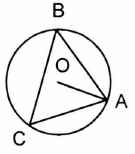Options:

A. 40 degree

B. 50 degree

C. 70 degree

D. 80 degree

JSTSE Previous Year Question Papers 2010-11 Ques No 10:

If P and Q are any two points lying on the sides DC and AB respectively of a parallelogram ABCD, then the ratio of ar(∆APB) to ar(∆BQC) is

Options:

A. 2 : 3

B. 1 : 2

C. 2 : 1

D. 1 : 1

JSTSE Previous Year Question Papers 2010-11 Ques No 11:

In figure, four points A, B, C, D are on a circle. AC and BD intersect at E such that ∠BEC = 130 and ∠ECD = 20 then ∠BAC is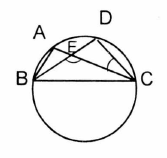Options:

A. 50 degree

B. 80 degree

C. 110 degree

D. 150 degree

JSTSE Previous Year Question Paper 2010-11 Ques No 12:

In figure, 2r is the radius of circle, then the shaded area of the region isOptions:

A. πr2/2

B. 2πr2/3

C. πr2

D. 3πr2/2

JSTSE Previous Year Question Papers 2010-11 Ques No 13:

The radius and slant height of a cone are in the ratio 4 : 7. If the curved surface area is 792 cm2, then radius will be

Options:

A. 7 cm

B. 11 cm

C. 12 cm

D. 28 cm

JSTSE Previous Year Question Papers 2010-11 Ques No 14:

If D, E, F are mid points of sides BC, CA and AB respectively of a triangle ABC, then the ratio of ar(ABDE) and ar(ABC) is

Options:

A. 1 : 2

B. 2 : 3

C. 3 : 4

D. none of above

JSTSE Previous Year Question Paper 2010-11 Ques No 15:

The diameter of a sphere is decreased by 25% then the ratio of its curved surface area to that of new sphere is

Options:

A. 4 : 3

B. 12 : 11

C. 16 : 9

D. 32 : 27

JSTSE Previous Year Question Papers 2010-11 Ques No 16:

The distance between points A(2, 0) and B(6, 3) is

Options:

A. 7 units

B. 6 units

C. 5 units

D. 4 units

JSTSE Previous Year Question Paper 2010-11 Ques No 17:

The volume of the sphere curved out of a cube of side 7 cm is

Options:

A. 343π /6 cm3

B. 343π /7 cm3

C. 343π /8 cm3

D. None of above

JSTSE Previous Year Question Papers 2010-11 Ques No 18:

If M is the mean of x1, x2, x3, …, x10, then the value of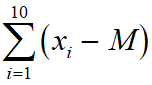Options:

A. 10

B. 5

C. 15

D. 0

JSTSE Previous Year Question Paper 2010-11 Ques No 19:

The sum of deviations of a set of n values of x1, x2, x3, …, xn measured from 15 and -3 are -90 and 54 respectively. Then the value of n is

Options:

A. 12

B. 8

C. 17

D. 6

JSTSE Previous Year Question Papers 2010-11 Ques No 20:

If the medians of numbers 29, 32, 48, 50, x, x+2, 72, 78, 84, 95 is 63, then the value of x is

Options:

A. 52

B. 56

C. 60

D. 62

JSTSE Previous Year Question Paper 2010-11 Ques No 21:

In a cricket match, a batsman hits a boundary 6 times out of 30 balls he plays, then the probability that he does not hit a boundary is

Options:

A. 4/5

B. 3/5

C. 2/5

D. none of above

JSTSE Previous Year Question Papers 2010-11 Ques No 22:

In the figure below, the value of ∠BOC, if AB || CD, is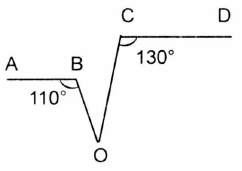Options:

A. 50 degree

B. 60 degree

C. 70 degree

D. 120 degree

JSTSE Previous Year Question Paper 2010-11 Ques No 23:

The value of tan1tan2tan3….tan89 is

Options:

A. 1

B. -1

C. 0

D. None of these

JSTSE Previous Year Question Papers 2010-11 Ques No 24:

If asinθ = bcosθ, then the value of sec2θ is

Options:

A. a2/b2

B. b2/a2

C. b2/(a2 + b2)

D. (a2 + b2)/a2

JSTSE Previous Year Question Paper 2010-11 Ques No 25:

If both (x – 2) and (x – 1/2) are factors of px2 + 5x + r, then

Options:

A. p = r

B. p > r

C. p < r

D. None of these

JSTSE
Previous Year Question Papers
2007-082008-092009-102010-112011-12
2012-132013-142014-152015-162016-17
2018-19PhysicsChemistryMathsBiology
Get Online Maths Tuition by AMBiPi for Class 8 to 12